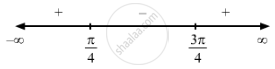Share

F(X) = Sin 2x, 0<X< π . - CBSE (Arts) Class 12 - Mathematics

Question

f(x) = sin 2x, 0 < x < $\pi$ .

Solution

$\text { Given }: \hspace{0.167em} f\left( x \right) = \sin 2x$

$\Rightarrow f'\left( x \right) = 2 \cos 2x$

$\text { For a local maximum or a local minimum, we must have }$

$f'\left( x \right) = 0$

$\Rightarrow 2 \cos 2x = 0$

$\Rightarrow \cos 2x = 0$

$\Rightarrow x = \frac{\pi}{4} or \frac{3\pi}{4}$Sincef '(x) changes from positive to negative when x increases through $\frac{\pi}{4}$, x = $\frac{\pi}{4}$ is the point of maxima.
The local maximum value of  f (x) at x = $\frac{\pi}{4}$ is given by $\sin\left( \frac{\pi}{2} \right) = 1$

Sincef '(x) changes from negative to positive when x increases through

$\frac{3\pi}{4}$ x = $\frac{3\pi}{4}$ is the point of minima.
The local minimum value of  f (x) at x = $\frac{3\pi}{4}$ is given by $\sin\left( \frac{3\pi}{2} \right) = - 1$
Is there an error in this question or solution?

Video TutorialsVIEW ALL 

Solution F(X) = Sin 2x, 0<X< π . Concept: Graph of Maxima and Minima.
S